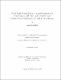## Novel single-source surface integral equations for scattering on 2-D penetrable cylinders and current flow modeling in 2-D and 3-D conductors##### Date
2012-06, 2012-05, 2013-01
Menshov, Anton
IEEE
##### Abstract
Accurate modeling of current flow and network parameter extraction in 2-D and 3-D conductors has an important application in signal integrity of high-speed interconnects. In this thesis, we propose a new rigorous single-source Surface-Volume-Surface Electric Field Integral Equation (SVS-EFIE) for magnetostatic analysis of 2-D transmission lines and broadband resistance and inductance extraction in 3-D interconnects. Furthermore, the novel integral equation can be used for the solution of full-wave scattering problems on penetrable 2-D cylinders of arbitrary cross-section under transverse magnetic polarization. The new integral equation is derived from the classical Volume Electric Field Integral Equation (V-EFIE) by representing the electric field inside a conductor or a scatterer as a superposition of the cylindrical waves emanating from the conductor’s surface. This converts the V-EFIE into a surface integral equation involving only a single unknown function on the surface. The novel equation features a product of integral operators mapping the field from the conductor surface to its volume and back to its surface terming the new equation the Surface-Volume-Surface EFIE. The number of unknowns in the proposed SVS-EFIE is approximately the square root of the number of degrees of freedom in the traditional V-EFIE; therefore, it allows for substantially faster network parameter extraction and solutions to 2-D scattering problems without compromising the accuracy. The validation and benchmark of the numerical implementation of the Method of Moment discretization of the novel SVS-EFIE has been done via comparisons against numerical results obtained by using alternative integral equations, data found in literature, simulation results acquired from the CAD software, and analytic formulas.
##### Keywords
computational electromagnetics, network parameter extraction, current flow modeling, scattering problems, integral equations, resistance and inductance
##### Citation
A. Menshov and V. Okhmatovski, “Novel surface integral equation formulation for accurate broadband RL extraction in transmission lines of arbitrary cross-section,” Microwave Symposium Digest (MTT), 2012 IEEE MTT-S International, pp. 1-3, 17–22 June 2012.
A. Menshov and V. Okhmatovski, “Method of moment solution of Surface-Volume-Surface Electric Field Integral Equation for two-dimensional transmission lines of complex cross-sections,” Signal and Power Integrity (SPI), 2012 IEEE 16th Workshop on, pp. 31–34, 13–16 May 2012.
A. Menshov and V. Okhmatovski, “New Single-Source Surface Integral Equations for Scattering on Penetrable Cylinders and Current Flow Modeling in 2-D Conductors,” IEEE Trans. Microw. Theory Techn., Vol. 61, No. 1, pp. 341-350, Jan. 2013.